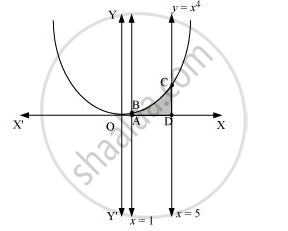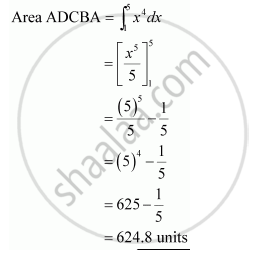Share

# Find the Area Under the Given Curves and Given Lines: Y = X4, X = 1, X = 5 And X –Axis - Mathematics

#### Question

Find the area under the given curves and given lines:

y = x4x = 1, x = 5 and x –axis

#### SolutionIs there an error in this question or solution?